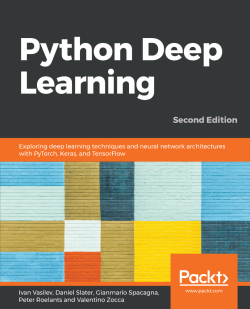# Python Deep Learning - Second Edition4.7 (3 reviews total)
By Ivan Vasilev , Daniel Slater , Gianmario Spacagna and 2 more
• \$28.99 eBook
• \$41.99 Print + eBook
What do you get with a Packt Subscription?

• Instant access to this title and 7,500+ eBooks & Videos
• Constantly updated with 100+ new titles each month
• Breadth and depth in over 1,000+ technologies

With the surge in artificial intelligence in applications catering to both business and consumer needs, deep learning is more important than ever for meeting current and future market demands. With this book, you’ll explore deep learning, and learn how to put machine learning to use in your projects.

This second edition of Python Deep Learning will get you up to speed with deep learning, deep neural networks, and how to train them with high-performance algorithms and popular Python frameworks. You’ll uncover different neural network architectures, such as convolutional networks, recurrent neural networks, long short-term memory (LSTM) networks, and capsule networks. You’ll also learn how to solve problems in the fields of computer vision, natural language processing (NLP), and speech recognition. You'll study generative model approaches such as variational autoencoders and Generative Adversarial Networks (GANs) to generate images. As you delve into newly evolved areas of reinforcement learning, you’ll gain an understanding of state-of-the-art algorithms that are the main components behind popular games Go, Atari, and Dota.

By the end of the book, you will be well-versed with the theory of deep learning along with its real-world applications.

Publication date:
January 2019
Publisher
Packt
Pages
386
ISBN
9781789348460

# Machine Learning - an Introduction

"Machine Learning (CS229) is the most popular course at Stanford. Why? Because, increasingly, machine learning is eating the world." - Laura Hamilton, Forbes

Machine learning(ML) techniques are being applied in a variety of fields, and data scientists are being sought after in many different industries. With machine learning, we identify the processes through which we gain knowledge that is not readily apparent from data in order to make decisions. Applications of machine learning techniques may vary greatly, and are found in disciplines as diverse as medicine, finance, and advertising.

In this chapter, we'll present different machine learning approaches, techniques, some of their applications to real-world problems, and we'll also introduce one of the major open source packages available in Python for machine learning, PyTorch. This will lay the foundation for the later chapters in which we'll focus on a particular type of machine learning approach using neural networks, which will aim to emulate brain functionality. In particular, we will focus on deep learning. Deep learning makes use of more advanced neural networks than those used during the 1980s. This is not only a result of recent developments in the theory, but also advancements in computer hardware. This chapter will summarize what machine learning is and what it can do, preparing the reader to better understand how deep learning differentiates itself from popular traditional machine learning techniques.

This chapter will cover the following topics:

• Introduction to machine learning
• Different machine learning approaches
• Neural networks
• Introduction to PyTorch

# Introduction to machine learning

Machine learning is often associated with terms such as big data and artificial intelligence (AI). However, both are quite different to machine learning. In order to understand what machine learning is and why it's useful, it's important to understand what big data is and how machine learning applies to it.

Big data is a term used to describe huge datasets that are created as the result of large increases in data that is gathered and stored. For example, this may be through cameras, sensors, or internet social sites.

It's estimated that Google alone processes over 20 petabytes of information per day, and this number is only going to increase. IBM estimated that every day, 2.5 quintillion bytes of data is created, and that 90% of all the data in the world has been created in the last two years (

Clearly, humans alone are unable to grasp, let alone analyze, such huge amounts of data, and machine learning techniques are used to make sense of these very large datasets. Machine learning is the tool used for large-scale data processing. It is well-suited to complex datasets that have huge numbers of variables and features. One of the strengths of many machine learning techniques, and deep learning in particular, is that they perform best when used on large datasets, thus improving their analytic and predictive power. In other words, machine learning techniques, and deep learning neural networks in particular, learn best when they can access large datasets where they can discover patterns and regularities hidden in the data.

On the other hand, machine learning's predictive ability can be successfully adapted to artificial intelligence systems. Machine learning can be thought of as the brain of an AI system. AI can be defined (though this definition may not be unique) as a system that can interact with its environment. Also, AI machines are endowed with sensors that enable them to know the environment they are in, and tools with which they can relate back to the environment. Machine learning is therefore the brain that allows the machine to analyze the data ingested through its sensors to formulate an appropriate answer. A simple example is Siri on an iPhone. Siri hears the command through its microphone and outputs an answer through its speakers or its display, but to do so, it needs to understand what it's being told. Similarly, driverless cars will be equipped with cameras, GPS systems, sonars, and LiDAR, but all this information needs to be processed in order to provide a correct answer. This may include whether to accelerate, brake, or turn. Machine learning is the information-processing method that leads to the answer.

We explained what machine learning is, but what about deep learning (DL)? For now, let's just say that deep learning is a subfield of machine learning. DL methods share some special common features. The most popular representatives of such methods are deep neural networks.

# Different machine learning approaches

As we have seen, the term machine learning is used in a very general way, and refers to the general techniques used to extrapolate patterns from large sets, or it is the ability to make predictions on new data based on what is learned by analyzing available known data. Machine learning techniques can roughly be divided in two large classes, while one more class is often added. Here are the classes:

• Supervised learning
• Unsupervised learning
• Reinforcement learning

# Supervised learning

Supervised learning algorithms are a class of machine learning algorithms that use previously-labeled data to learn its features, so they can classify similar but unlabeled data. Let's use an example to understand this concept better.

Let's assume that a user receives a large amount of emails every day, some of which are important business emails and some of which are unsolicited junk emails, also known as spam. A supervised machine algorithm will be presented with a large body of emails that have already been labeled by a teacher as spam or not spam (this is called training data). For each sample, the machine will try to predict whether the email is spam or not, and it will compare the prediction with the original target label. If the prediction differs from the target, the machine will adjust its internal parameters in such a way that the next time it encounters this sample it will classify it correctly. Conversely, if the prediction was correct, the parameters will stay the same. The more training data we feed to the algorithm, the better it becomes (this rule has caveats, as we'll see next).

In the example we used, the emails had only two classes (spam or not spam), but the same principles apply for tasks with arbitrary numbers of classes. For example, we could train the software on a set of labeled emails where the classes are Personal, Business/Work, Social, or Spam.

In fact, Gmail, the free email service by Google, allows the user to select up to five categories, which are labeled as the following:

• Primary: Includes person-to-person conversations
• Social: Includes messages from social networks and media-sharing sites
• Promotions: Includes marketing emails, offers, and discounts
• Updates: Includes bills, bank statements, and receipts
• Forums: Includes messages from online groups and mailing lists

In some cases, the outcome may not necessarily be discrete, and we may not have a finite number of classes to classify our data into. For example, we may try to predict the life expectancy of a group of people based on their predetermined health parameters. In this case, the outcome is a continuous function, that is, the number years the person is expected to live, and we don't talk about classification but rather regression.

One way to think of supervised learning is to imagine we are building a function, f, defined over a dataset, which comprises information organized by features. In the case of email classification, the features can be specific words that may appear more frequently than others in spam emails. The use of explicit sex-related words will most likely identify a spam email rather than a business/work email. On the contrary, words such as meeting, business, or presentation are more likely to describe a work email. If we have access to metadata, we may also use the sender's information as a feature. Each email will then have an associated set of features, and each feature will have a value (in this case, how many times the specific word is present in the email body). The machine learning algorithm will then seek to map those values to a discrete range that represents the set of classes, or a real value in the case of regression. The definition of the f function is as follows:In later chapters, we'll see several examples of either classification or regression problems. One such problem we'll discuss is the classification of handwritten digits (the famous Modified National Institute of Standards and Technology, or MNIST, database). When given a set of images representing 0 to 9, the machine learning algorithm will try to classify each image in one of the 10 classes, wherein each class corresponds to one of the 10 digits. Each image is 28x28 (= 784) pixels in size. If we think of each pixel as one feature, then the algorithm will use a 784-dimensional feature space to classify the digits.

The following screenshot depicts the handwritten digits from the MNIST dataset: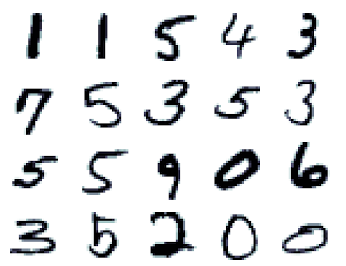Example of handwritten digits from the MNIST dataset

In the next sections, we'll talk about some of the most popular classical supervised algorithms. The following is by no means an exhaustive list or a thorough description of each machine learning method. We can refer to the book Python Machine Learning by Sebastian Raschka (https://www.packtpub.com/big-data-and-business-intelligence/python-machine-learning). It's a simple review meant to provide the reader with a flavor of the different techniques. Also, at the end of this chapter in the Neural networks section, we'll introduce neural networks and we'll talk about how deep learning differs from the classical machine learning techniques.

# Linear and logistic regression

Regression algorithms are a type of supervised algorithm that uses features of the input data to predict a value, such as the cost of a house, given certain features, such as size, age, number of bathrooms, number of floors, and location. Regression analysis tries to find the value of the parameters for the function that best fits an input dataset.

In a linear-regression algorithm, the goal is to minimize a cost function by finding appropriate parameters for the function, over the input data that best approximates the target values. A cost function is a function of the error, that is, how far we are from getting a correct result. A popular cost function is the mean square error (MSE), where we take the square of the difference between the expected value and the predicted result. The sum over all the input examples gives us the error of the algorithm and represents the cost function.

Say we have a 100-square-meter house that was built 25 years ago with 3 bathrooms and 2 floors. Let's also assume that the city is divided into 10 different neighborhoods, which we'll denote with integers from 1 to 10, and say this house is located in the area denoted by 7. We can parameterize this house with a five-dimensional vector, x = (100, 25, 3, 2, 7). Say that we also know that this house has an estimated value of €100,000. What we want is to create a function, f, such that f(x) = 100000.

In linear regression, this means finding a vector of weights, w= (w1, w2, w3, w4, w5), such that the dot product of the vectors, x • w = 10000, would be 100*w1 + 25*w2 + 3*w3 + 2*w4 + 7*w5 = 100000 or. If we had 1,000 houses, we could repeat the same process for every house, and ideally we would like to find a single vector, w, that can predict the correct value that is close enough for every house. The most common way to train a linear regression model can be seen in the following pseudocode block:

`Initialize the vector w with some random valuesrepeat:   E = 0 # initialize the cost function E with 0    for every sample/target pair (xi, ti) of the training set:        E +=# here ti is the real cost of the house    MSE = E / total_number_of_samples # Mean Square Error    use gradient descent to update the weights w based on MSE  until MSE falls below threshold`

First, we iterate over the training data to compute the cost function, MSE. Once we know the value of MSE, we'll use the gradient-descent algorithm to update w. To do this, we'll calculate the derivatives of the cost function with respect to each weight, wi . In this way, we'll know how the cost function changes (increase or decrease) with respect to wi . Then we'll update its value accordingly. In Chapter 2, Neural Networks, we will see that training neural networks and linear/logistic regressions have a lot in common.

We demonstrated how to solve a regression problem with linear regression. Let's take another task: trying to determine whether a house is overvalued or undervalued. In this case, the target data would be categorical [1, 0] - 1 for overvalued, 0 for undervalued, if the price of the house will be an input parameter instead of target value as before. To solve the task, we'll use logistic regression. This is similar to linear regression but with one difference: in linear regression, the output is. However, here the output will be a special logistic function (https://en.wikipedia.org/wiki/Logistic_function),. This will squash the value ofin the (0:1) interval. You can think of the logistic function as a probability, and the closer the result is to 1, the more chance there is that the house is overvalued, and vice versa. Training is the same as with linear regression, but the output of the function is in the (0:1) interval and the labels is either 0 or 1.

Logistic regression is not a classification algorithm, but we can turn it into one. We just have to introduce a rule that determines the class based on the logistic function output. For example, we can say that a house is overvalued if the value ofand undervalued otherwise.

# Support vector machines

A support vector machine (SVM) is a supervised machine learning algorithm that is mainly used for classification. It is the most popular member of the kernel method class of algorithms. An SVM tries to find a hyperplane, which separates the samples in the dataset.

A hyperplane is a plane in a high-dimensional space. For example, a hyperplane in a one-dimensional space is a point, and in a two-dimensional space, it would just be a line. We can think of classification as a process of trying to find a hyperplane that will separate different groups of data points. Once we have defined our features, every sample (in our case, an email) in the dataset can be thought of as a point in the multidimensional space of features. One dimension of that space represents all the possible values of one feature. The coordinates of a point (a sample) are the specific values of each feature for that sample. The ML algorithm task will be to draw a hyperplane to separate points with different classes. In our case, the hyperplane would separate spam from non-spam emails.

In the following diagram, on the top and bottom, you can see two classes of points (red and blue) that are in a two-dimensional feature space (the x and y axes). If both the x and y values of a point are below five, then the point is blue. In all other cases, the point is red. In this case, the classes are linearly-separable, meaning we can separate them with a hyperplane. Conversely, the classes in the image at the bottom are linearly-inseparable: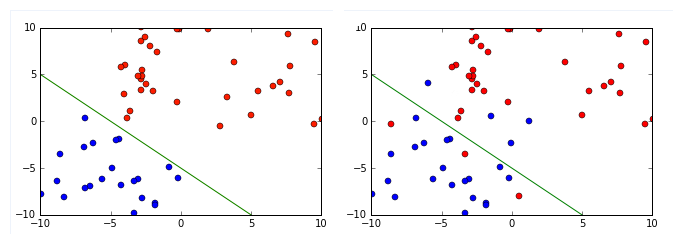The SVM tries to find a hyperplane that maximizes the distance between itself and the points. In other words, from all possible hyperplanes that can separate the samples, the SVM finds the one that has the maximum distance from all points. In addition, SVMs can also deal with data that is not linearly-separable. There are two methods for this: introducing soft margins or using the kernel trick.

Soft margins work by allowing a few misclassified elements while retaining the most predictive ability of the algorithm. In practice, it's better not to overfit the machine learning model, and we could do so by relaxing some of the support-vector-machine hypotheses.

The kernel trick solves the same problem in a different way. Imagine that we have a two-dimensional feature space, but the classes are linearly-inseparable. The kernel trick uses a kernel function that transforms the data by adding more dimensions to it. In our case, after the transformation, the data will be three-dimensional. The linearly-inseparable classes in the two-dimensional space will become linearly-separable in the three dimensions and our problem is solved: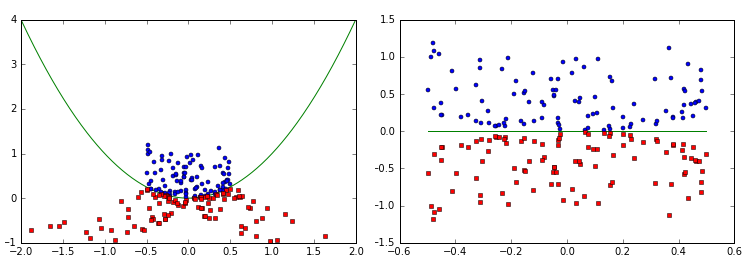In the graph on the left image, we can see a non-linearly-separable set before the kernel was applied and on the bottom. On the right, we can see the same dataset after the kernel has been applied, and the data can be linearly separated

# Decision Trees

Another popular supervised algorithm is the decision tree. A decision tree creates a classifier in the form of a tree. This is composed of decision nodes, where tests on specific attributes are performed; and leaf nodes, which indicate the value of the target attribute. To classify a new sample, we start at the root of the tree and navigate down the nodes until we reach a leaf.

A classic application of this algorithm is the Iris flower dataset (http://archive.ics.uci.edu/ml/datasets/Iris), which contains data from 50 samples of three types of Irises (Iris Setosa, Iris Virginica, and Iris Versicolor). Ronald Fisher, who created the dataset, measured four different features of these flowers:

• The length of their sepals
• The width of their sepals
• The length of their petals
• The width of their petals

Based on the different combinations of these features, it's possible to create a decision tree to decide which species each flower belongs to. In the following diagram, we have defined a decision tree that will correctly classify almost all the flowers using only two of these features, the petal length and width: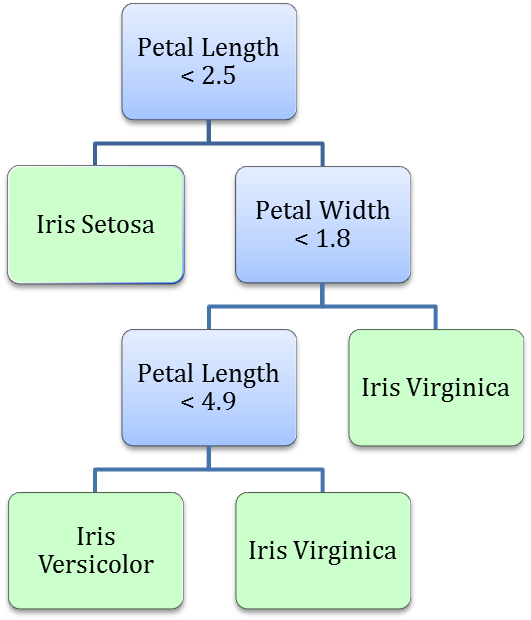To classify a new sample, we start at the root note of the tree (petal length). If the sample satisfies the condition, we go left to the leaf, representing the Iris Setosa class. If not, we go right to a new node (petal width). This process continues until we reach a leaf. There are different ways to build decision trees, and we will discuss them later, in the chapter.

In recent years, decision trees have seen two major improvements. The first is Random Forests, which is an ensemble method that combines the predictions of multiple trees. The second is Gradient-Boosting Machines, which creates multiple sequential decision trees, where each tree tries to improve the errors made by the previous tree. Thanks to these improvements, decision trees have become very popular when working with certain types of data. For example, they are one of the most popular algorithms used in Kaggle competitions.

# Naive Bayes

Naive Bayes is different from many other machine learning algorithms. Most machine learning techniques try to evaluate the probability of a certain event, Y , and given conditions, X, which we denote with. For example, when we are given a picture that represents digits (that is, a picture with a certain distribution of pixels), what is the probability that the number is five? If the pixel's distribution is close to the pixel distribution of other examples that were labeled as five, the probability of that event will be high. If not, the probability will be low.

Sometimes we have the opposite information, given the fact that we know that we have an event, Y. We also know the probability, that our sample is X. The Bayes theorem states that, wheremeans the probability of event, X, given Y, which is also why naive Bayes is called a generative approach. For example, we may calculate the probability that a certain pixel configuration represents the number five, knowing what the probability is. Given that we have a five, that a random pixel configuration may match the given one.

This is best understood in the realm of medical testing. Let's say we conduct a test for a specific disease or cancer. Here, we want to know the probability of a patient having a particular disease, given that our test result was positive. Most tests have a reliability value, which is the percentage chance of the test being positive when administered on people with a particular disease. By reversing theexpression, we get the following:

p(cancer | test=positive) = p(test=positive | cancer) * p(cancer) / p(test=positive)

Let's assume that the test is 98% reliable. This means that if the test is positive, it will also be positive in 98% of cases. Conversely, if the person does not have cancer, the test result will be negative. Let's make some assumptions on this kind of cancer:

• This particular kind of cancer only affects older people
• Only 2% of people under 50 have this kind of cancer
• The test administered on people under 50 is positive only for 3.9% of the population (we could have derived this fact from the data, but we provide this information for the purpose of simplicity)

We can ask the following question: if a test is 98% accurate for cancer and if a 45-year-old person took the test, which turned out to be positive, what is the probability that they may have cancer? Using the preceding formula, we can calculate the following:

p(cancer | test=positive) = 0.98 * 0.02 / 0.039 = 0.50

We call this classifier naive because it assumes the independence of different events to calculate their probability. For example, if the person had two tests instead of one, the classifier will assume that the outcome of test 2 did not know about the outcome of test 1, and the two tests were independent from one another. This means that taking test 1 could not change the outcome of test 2, and therefore its result was not biased by the first test.

# Unsupervised learning

The second class of machine learning algorithms is unsupervised learning. Here, we don't label the data beforehand, but instead we let the algorithm come to its conclusion. One of the most common, and perhaps simplest, examples of unsupervised learning is clustering. This is a technique that attempts to separate the data into subsets.

To illustrate this, let's view the spam-or-not-spam email classification as an unsupervised learning problem. In the supervised case, for each email, we had a set of features and a label (spam or not spam). Here, we'll use the same set of features, but the emails will not be labeled. Instead, we'll ask the algorithm, when given the set of features, to put each sample in one of two separate groups (or clusters). Then the algorithm will try to combine the samples in such a way that the intraclass similarity (which is the similarity between samples in the same cluster) is high and the similarity between different clusters is low. Different clustering algorithms use different metrics to measure similarity. For some more advanced algorithms, you don't have to specify the number of clusters.

In the following graph, we show how a set of points can be classified to form three subsets: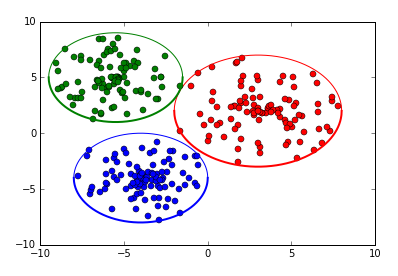Deep learning also uses unsupervised techniques, albeit different than clustering. In natural language processing (NLP), we use unsupervised (or semi-supervised, depending on who you ask) algorithms for vector representations of words. The most popular way to do this is called word2vec. For each word, we use its surrounding words (or its context) in the text and feed them to a simple neural network. The network produces a numerical vector, which contains a lot of information for the word (derived by the context). We then use these vectors instead of the words for various NLP tasks, such as sentiment analysis or machine translation. We’ll describe word2vec in Chapter 7, Recurrent Neural Networks and Language Models.

Another interesting application of unsupervised learning is in generative models, as opposed to discriminative models. We will train a generative model with a large amount of data of a certain domain, such as images or text, and the model will try to generate new data similar to the one we used for training. For example, a generative model can colorize black and white images, change facial expressions in images, or even synthesize images based on a text description. In Chapter 6, Generating Images with GANs and Variational Autoencoders, we'll look at two of the most popular generative techniques, Variational Autoencoders and Generative Adversarial Networks (GANs).

The following depicts the techniques: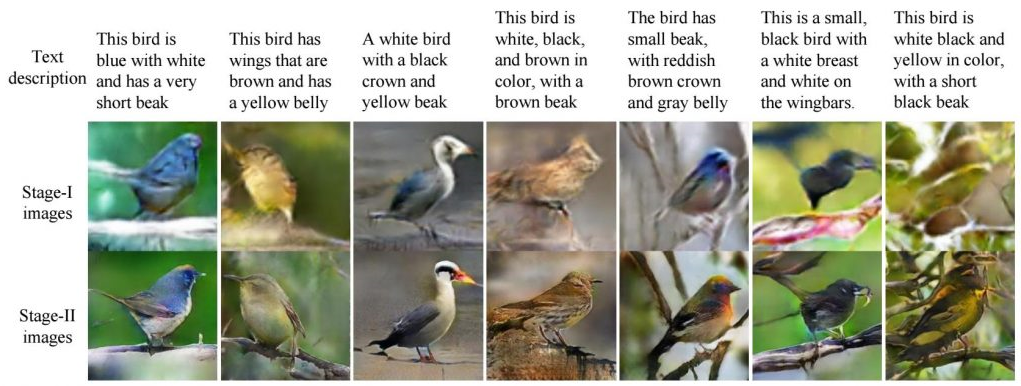In the preceding image, you can see how the authors of StackGAN: Text to Photo-realistic Image Synthesis with Stacked Generative Adversarial Networks, Han Zhang, Tao Xu, Hongsheng Li, Shaoting Zhang, Xiaogang Wang, Xiaolei Huang, and Dimitris Metaxas, use a combination of supervised learning and unsupervised GANs to produce photo-realistic images based on text descriptions.

# K-means

K-means is a clustering algorithm that groups the elements of a dataset into k distinct clusters (hence the k in the name). Here is how it works:

1. Choose k random points, called centroids, from the feature space, which will represent the center of each of the k clusters.
2. Assign each sample of the dataset (that is, each point in the feature space) to the cluster with the closest centroid.
3. For each cluster, we recomputed new centroids by taking the mean values of all the points in the cluster.
4. With the new centroids, we repeat steps 2 and 3 until the stopping criteria is met.

The preceding method is sensitive to the initial choice of random centroids and it may be a good idea to repeat it with different initial choices. It's also possible for some centroids to not be close to any of the points in the dataset, reducing the number of clusters down from k. Finally, it's worth mentioning that if we used k-means with k=3 on the Iris dataset, we may get different distributions of the samples compared to the distribution of the decision tree that we'd introduced. Once more, this highlights how important it is to carefully choose and use the correct machine learning method for each problem.

Now let's discuss a practical example that uses k-means clustering. Let's say a pizza-delivery place wants to open four new franchises in a city, and they need to choose the locations for the sites. We can solve this problem with k-means:

1. Find the locations where pizza is ordered from most often and these will be our data points.
2. Choose four random points where the site locations will be located.
1. By using k-means clustering, we can identify the four best locations that minimize the distance to each delivery place: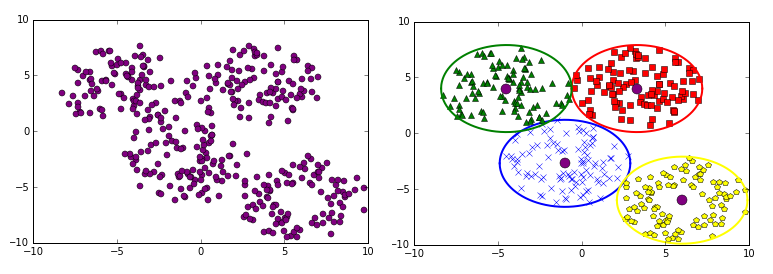In the left image, we can see the distribution of points where pizza is delivered most often. The round pints in the right image indicate where the new franchises should be located and their corresponding delivery areas

# Reinforcement learning

The third class of machine learning techniques is called reinforcement learning (RL). We will illustrate this with one of the most popular applications of reinforcement learning: teaching machines how to play games. The machine (or agent) interacts with the game (or environment). The goal of the agent is to win the game. To do this, the agent takes actions that can change the environment’s state. The environment provides the agent with reward signals that help the agent to decide its next action. Winning the game would provide the biggest reward. In formal terms, the goal of the agent is to maximize the total rewards it receives throughout the game: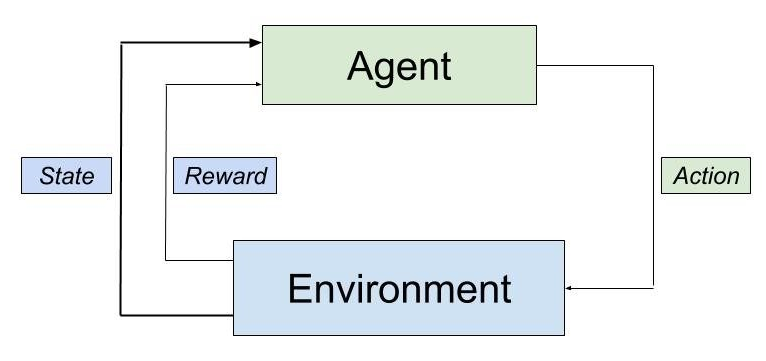The interaction of different elements of a reinforcement learning system

In reinforcement learning, the agent takes an action, which changes the state of the environment. The agent uses the new state and the reward to determine its next action.

Let’s imagine a game of chess as an RL problem. The environment here would include the chess board along with the locations of the pieces. The goal of our agent is to beat the opponent. The agent will then receive a reward when they capture the opponent’s piece, and they will win the biggest reward if they checkmate the opponent. Conversely, if the opponent captures a piece or checkmates the agent, the reward will be negative. However, as part of their larger strategies, the players will have to make moves that neither capture a piece, nor checkmate the other’s king. The agent won’t receive any reward then. If this was a supervised learning problem, we would have to provide a label or a reward for each move. This is not the case with reinforcement learning. In this book, we’ll demonstrate how to use RL to allow the agent to use its previous experience in order to take new actions and learn from them in situations such as this.

Let’s take another example, in which sometimes we have to sacrifice a pawn to achieve a more important goal (such as a better position on the chessboard). In such situations, our humble agent has to be smart enough to take a short-term loss as a long-term gain. In an even more extreme case, imagine we had the bad luck of playing against Magnus Carlsen, the current world chess champion. Surely, the agent will lose in this case. However, how would we know which moves were wrong and led to the agent's loss? Chess belongs to a class of problems where the game should be considered in its entirety in order to reach a successful solution, rather than just looking at the immediate consequences of each action. Reinforcement learning will give us the framework that will help the agent to navigate and learn in this complex environment.

An interesting problem arises from this newfound freedom to take actions. Imagine that the agent has learned one successful chess-playing strategy (or policy, in RL terms). After some games, the opponent might guess what that policy is and manage to beat us. The agent will now face a dilemma with the following decisions: either to follow the current policy and risk becoming predictable, or to experiment with new moves that will surprise the opponent, but also carry the risk of turning out even worse. In general terms, the agent uses a policy that gives them a certain reward, but their ultimate goal is to maximize the total reward. A modified policy might be more rewarding and the agent will be ineffective if they don’t try to find such a policy. One of the challenges of reinforcement learning is the tradeoff between exploitation (following the current policy) and exploration (trying new moves). In this book, we’ll learn the strategies to find the right balance between the two. We’ll also learn how to combine deep neural networks with reinforcement learning, which made the field so popular in recent years.

So far, we’ve used only games as examples; however, many problems can fall into the RL domain. For example, you can think of an autonomous vehicle driving as an RL problem. The vehicle can get positive rewards if it stays within its lane and observes the traffic rules. It will gain negative rewards if it crashes. Another interesting recent application of RL is in managing stock portfolios. The goal of the agent would be to maximize the portfolio value. The reward is directly derived from the value of the stocks in the portfolio.

# Q-learning

Q-learning is an off-policy temporal-difference reinforcement learning algorithm. What a mouthful! But fear not, let’s not worry about what all this means, and instead just see how the algorithm works. To do this, we’ll use the game of chess we introduced in the previous section. As a reminder, the board configuration (the locations of the pieces) is the current state of the environment. Here, the agents can take actions, a, by moving pieces, thus changing the state into a new one. We'll represent a game of chess as a graph where the different board configurations are the graph’s vertices, and the possible moves from each configuration are the edges. To make a move, the agent follows the edge from the current state, s, to a new state, s'. The basic Q-learning algorithm uses Q-table to help the agent decide which moves to make. The Q-table contains one row for each board configuration, while the columns of the table are all possible actions that the agent can take (the moves). A table cell, q(s, a), contains the cumulative expected reward, called Q-value. This is the potential total reward that the agent will receive for the remainder of the game if they take an action, a, from their current state, s. At the beginning, the Q-table is initialized with an arbitrary value. With that knowledge, let’s see how Q-learning works:

`Initialize the Q table with some arbitrary valuefor each episode:    Observe the initial state s    for each step of the episode:        Select new action a using a policy based on the Q-table        Observe reward r and go to the new state s’        Update q(s, a) in the Q table using the Bellman Equation    until we reach a terminal state for the episode`

An episode starts with a random initial state and finishes when we reach the terminal state. In our case, one episode would be one full game of chess.

The question that arises is this: how does the agent's policy determine what will be the next action? To do so, the policy has to take into account the Q-values of all the possible actions from the current state. The higher the Q-value, the more attractive the action is. However, the policy will sometimes ignore the Q-table (exploitation of the existing knowledge) and choose another random action to find higher potential rewards (exploration). In the beginning, the agent will take random actions because the Q-table doesn’t contain much information. As time progresses and the Q-table is gradually filled, the agent will become more informed in interacting with the environment.

We update q(s, a) after each new action, by using Bellman equation. The Bellman equation is beyond the scope of this introduction, but we’ll discuss it in detail in the later chapters. For now, it's enough to know that the updated value, q(s, a), is based on the newly-received reward, r , as well as the maximum possible Q-value, q*(s’, a’), of the new state, s'.

This example was intended to help you understand the basic workings of Q-learning, but you might have noticed an issue with this. We store the combination of all possible board configurations and moves in the Q-table. This would make the table huge and impossible to fit in today’s computer memory. Fortunately, there is a solution for this: we can replace the Q-table with a neural network, which will tell the agent what the optimal action is in each state. In recent years, this development has allowed reinforcement learning algorithms to achieve superhuman performance on tasks such as the game of Go, Dota 2, and Doom. In this book, we’ll discuss how to apply Q-learning and other RL algorithms to some of these tasks.

# Components of an ML solution

So far, we've discussed three major classes of machine learning algorithms. However, to solve an ML problem, we'll need a system in which the ML algorithm is only part of it. The most important aspects of such a system are as follows:

• Learner: This is algorithm is used with its learning philosophy. The choice of this algorithm is determined by the problem we're trying to solve, since different problems can be better suited for certain machine learning algorithms.
• Training data: This is the raw dataset that we are interested in. This can be labeled or unlabeled. It's important to have enough sample data for the learner to understand the structure of the problem.
• Representation: This is how we express the data in terms of the chosen features, so that we can feed it to the learner. For example, to classify handwritten images of digits, we'll represent the image as an array of values, where each cell will contain the color value of one pixel. A good choice of representation of the data is important for achieving better results.
• Goal: This represents the reason to learn from the data for the problem at hand. This is strictly related to the target, and helps define how and what the learner should use and what representation to use. For example, the goal may be to clean our mailbox from unwanted emails, and this goal defines what the target of our learner is. In this case, it is the detection of spam emails.
• Target: This represents what is being learned as well as the final output. The target can be a classification of unlabeled data, a representation of input data according to hidden patterns or characteristics, a simulator for future predictions, or a response to an outside stimulus or strategy (in the case of reinforcement learning).

It can never be emphasized enough: any machine learning algorithm can only achieve an approximation of the target and not a perfect numerical description. Machine learning algorithms are not exact mathematical solutions to problems, they are just approximations. In the previous paragraph, we defined learning as a function from the space of features (the input) into a range of classes. We'll later see how certain machine learning algorithms, such as neural networks, can approximate any function to any degree, in theory. This theorem is called the Universal Approximation Theorem, but it does not imply that we can get a precise solution to our problem. In addition, solutions to the problem can be better achieved by better understanding the training data.

Typically, a problem that is solvable with classic machine learning techniques may require a thorough understanding and processing of the training data before deployment. The steps to solve an ML problem are as follows:

• Data collection: This implies the gathering of as much data as possible. In the case of supervised learning, this also includes correct labeling.
• Data processing: This implies cleaning the data, such as removing redundant or highly correlated features, or even filling missing data, and understanding the features that define the training data.
• Creation of the test case: Usually, the data can be divided into three sets:
• Training set: We use this set to train the ML algorithm.
• Validation set: We use this set to evaluate the accuracy of the algorithm with unknown data during training. We'll train the algorithm for some time on the training set and then we'll use the validation set to check its performance. If we are not satisfied with the result, we can tune the hyperparameters of the algorithm and repeat the process again. The validation set can also help us to determine when to stop the training. We'll learn more about this later in this section.
• Test set: When we finish tuning the algorithm with the training or validation cycle, we'll use the test set only once for a final evaluation. The test set is similar to the validation set in the sense that the algorithm hasn't used it during training. However, when we strive to improve the algorithm on the validation data, we may inadvertently introduce bias, which can skew the results in favor of the validation set and not reflect the actual performance. Because we use the test only once, this will provide a more objective measurement of the algorithm.
One of the reasons for the success of deep learning algorithms is that they usually require less data processing than classic methods. For a classic algorithm, you would have to apply different data processing and extract different features for each problem. With DL, you can apply the same data processing pipeline for most tasks. With DL, you can be more productive and you don't need as much domain knowledge for the task at hand compared to the classic ML algorithms.

There are many valid reasons to create testing and validation datasets. As mentioned, machine learning techniques can only produce an approximation of the desired result. Often, we can only include a finite and limited number of variables, and there may be many variables that are outside of our control. If we only used a single dataset, our model may end up memorizing the data, and producing an extremely high accuracy value on the data it has memorized. However, this result may not be reproducible on other similar but unknown datasets. One of the key goals of machine learning algorithms is their ability to generalize. This is why we create both, a validation set used for tuning our model selection during training, and a final test set only used at the end of the process to confirm the validity of the selected algorithm.

To understand the importance of selecting valid features and to avoid memorizing the data, which is also referred to as overfitting in the literature-and we'll use that term from now on-let's use a joke taken from an xkcd comic as an example (http://xkcd.com/1122):

"Up until 1996, no democratic US presidential candidate who was an incumbent and with no combat experience had ever beaten anyone whose first name was worth more in Scrabble."

It's apparent that such a rule is meaningless, but it underscores the importance of selecting valid features and the question, "how much is a name worth in Scrabble," can bear any relevance while selecting a US president? Also, this example doesn't have any predictive power over unknown data. We'll call this overfitting, which refers to making predictions that fit the data at hand perfectly, but don't generalize to larger datasets. Overfitting is the process of trying to make sense of what we'll call noise (information that does not have any real meaning) and trying to fit the model to small perturbations.

To further explain this, let's try to use machine learning to predict the trajectory of a ball thrown from the ground up into the air (not perpendicularly) until it reaches the ground again. Physics teaches us that the trajectory is shaped as a parabola. We also expect that a good machine learning algorithm observing thousands of such throws would come up with a parabola as a solution. However, if we were to zoom into the ball and observe the smallest fluctuations in the air due to turbulence, we might notice that the ball does not hold a steady trajectory but may be subject to small perturbations, which in this case is the noise. A machine learning algorithm that tries to model these small perturbations would fail to see the big picture and produce a result that is not satisfactory. In other words, overfitting is the process that makes the machine learning algorithm see the trees, but forgets about the forest: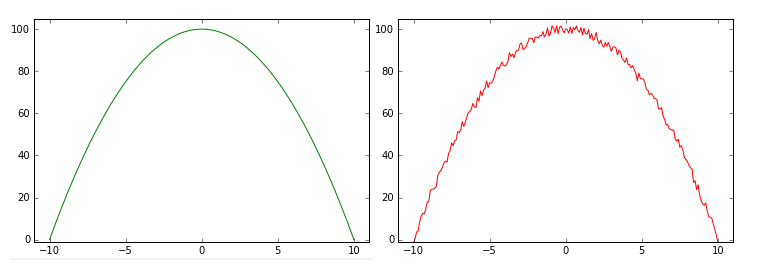A good prediction model versus a bad (overfitted) prediction model, with the trajectory of a ball thrown from the ground

This is why we separate the training data from the validation and test data; if the accuracy on the test data was not similar to the training data accuracy, that would be a good indication that the model overfits. We need to make sure that we don't make the opposite error either, that is, underfitting the model. In practice though, if we aim to make our prediction model as accurate as possible on our training data, underfitting is much less of a risk, and care is taken to avoid overfitting.

The following image depicts underfitting: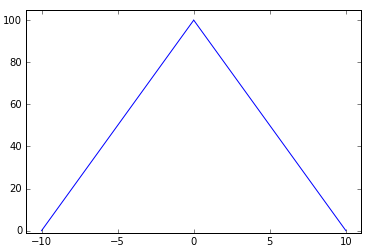Underfitting can be a problem as well

# Neural networks

In the previous sections, we introduced some of the popular classical machine learning algorithms. In this section, we'll talk about neural networks, which is the main focus of the book.

The first example of a neural network is called the perceptron, and this was invented by Frank Rosenblatt in 1957. The perceptron is a classification algorithm that is very similar to logistic regression. Such as logistic regression, it has weights, w, and its output is a function,, of the dot product,(orof the weights and input.

The only difference is that f is a simple step function, that is, if, then, or else, wherein we apply a similar logistic regression rule over the output of the logistic function. The perceptron is an example of a simple one-layer neural feedforward network: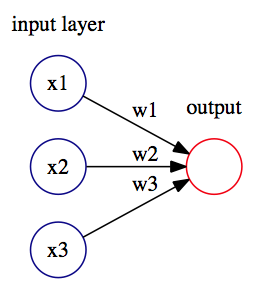A simple perceptron with three input units (neurons) and one output unit (neuron)

The perceptron was very promising, but it was soon discovered that is has serious limitations as it only works for linearly-separable classes. In 1969, Marvin Minsky and Seymour Papert demonstrated that it could not learn even a simple logical function such as XOR. This led to a significant decline in the interest in perceptron's.

However, other neural networks can solve this problem. A classic multilayer perceptron has multiple interconnected perceptron's, such as units that are organized in different sequential layers (input layer, one or more hidden layers, and an output layer). Each unit of a layer is connected to all units of the next layer. First, the information is presented to the input layer, then we use it to compute the output (or activation), yi, for each unit of the first hidden layer. We propagate forward, with the output as input for the next layers in the network (hence feedforward), and so on until we reach the output. The most common way to train neural networks is with a gradient descent in combination with backpropagation. We'll discuss this in detail in chapter 2, Neural Networks.

The following diagram depicts the neural network with one hidden layer: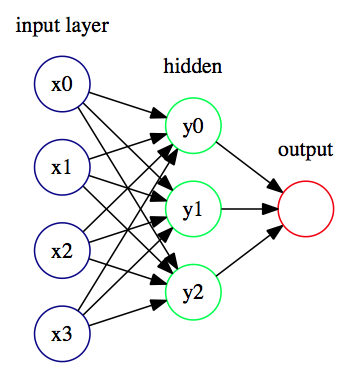Neural network with one hidden layer

Think of the hidden layers as an abstract representation of the input data. This is the way the neural network understands the features of the data with its own internal logic. However, neural networks are non-interpretable models. This means that if we observed the yi activations of the hidden layer, we wouldn't be able to understand them. For us, they are just a vector of numerical values. To bridge the gap between the network's representation and the actual data we're interested in, we need the output layer. You can think of this as a translator; we use it to understand the network's logic, and at the same time, we can convert it to the actual target values that we are interested in.

The Universal approximation theorem tells us that a feedforward network with one hidden layer can represent any function. It's good to know that there are no theoretical limits on networks with one hidden layer, but in practice we can achieve limited success with such architectures. In Chapter 3, Deep Learning Fundamentals, we'll discuss how to achieve better performance with deep neural networks, and their advantages over the shallow ones. For now, let's apply our knowledge by solving a simple classification task with a neural network.

# Introduction to PyTorch

In this section, we'll introduce PyTorch, version 1.0. PyTorch is an open source python deep learning framework, developed primarily by Facebook that has been gaining momentum recently. It provides the Graphics Processing Unit (GPU), an accelerated multidimensional array (or tensor) operation, and computational graphs, which we can be used to build neural networks. Throughout this book, we'll use PyTorch, TensorFlow, and Keras, and we'll talk in detail about these libraries and compare them in Chapter 3, Deep Learning Fundamentals.

The steps are as follows:

1. Let's create a simple neural network that will classify the Iris flower dataset. The following is the code block for creating a simple neural network:
`import pandas as pddataset = pd.read_csv('https://archive.ics.uci.edu/ml/machine-learning-databases/iris/iris.data',                      names=['sepal_length', 'sepal_width', 'petal_length', 'petal_width', 'species'])dataset['species'] = pd.Categorical(dataset['species']).codesdataset = dataset.sample(frac=1, random_state=1234)train_input = dataset.values[:120, :4]train_target = dataset.values[:120, 4]test_input = dataset.values[120:, :4]test_target = dataset.values[120:, 4]`
1. The preceding code is boilerplate code that downloads the Iris dataset CSV file and then loads it into the pandas DataFrame. We then shuffle the DataFrame rows and split the code into numpy arrays, train_input/train_target (flower properties/flower class), for the training data and test_input/test_target for the test data.
1. We'll use 120 samples for training and 30 for testing. If you are not familiar with pandas, think of this as an advanced version of NumPy. Let's define our first neural network:
`import torchtorch.manual_seed(1234)hidden_units = 5net = torch.nn.Sequential( torch.nn.Linear(4, hidden_units), torch.nn.ReLU(), torch.nn.Linear(hidden_units, 3))`
1. We'll use a feedforward network with one hidden layer with five units, a ReLU activation function (this is just another type of activation, defined simply as f(x) = max(0, x)), and an output layer with three units. The output layer has three units, whereas each unit corresponds to one of the three classes of Iris flower. We'll use one-hot encoding for the target data. This means that each class of the flower will be represented as an array (Iris Setosa = [1, 0, 0], Iris Versicolour = [0, 1, 0], and Iris Virginica = [0, 0, 1]), and one element of the array will be the target for one unit of the output layer. When the network classifies a new sample, we'll determine the class by taking the unit with the highest activation value.
2. torch.manual_seed(1234) enables us to use the same random data every time for the reproducibility of results.
3. Choose the optimizer and loss function:
`# choose optimizer and loss functioncriterion = torch.nn.CrossEntropyLoss()optimizer = torch.optim.SGD(net.parameters(), lr=0.1, momentum=0.9)`
1. With the criterion variable, we define the loss function that we'll use, in this case, this is cross-entropy loss. The loss function will measure how different the output of the network is compared to the target data.
1. We then define the stochastic gradient descent (SGD) optimizer with a learning rate of 0.1 and a momentum of 0.9. The SGD is a variation of the gradient descent algorithm. We'll discuss loss functions and SGD in detail in Chapter 2, Neural Networks. Now, let's train the network:
`# trainepochs = 50for epoch in range(epochs): inputs = torch.autograd.Variable(torch.Tensor(train_input).float()) targets = torch.autograd.Variable(torch.Tensor(train_target).long()) optimizer.zero_grad() out = net(inputs) loss = criterion(out, targets) loss.backward() optimizer.step() if epoch == 0 or (epoch + 1) % 10 == 0: print('Epoch %d Loss: %.4f' % (epoch + 1, loss.item()))`
1. We'll run the training for 50 epochs, which means that we'll iterate 50 times over the training dataset:
1. Create the torch variable that are input and target from the numpy array train_input and train_target.
2. Zero the gradients of the optimizer to prevent accumulation from the previous iterations. We feed the training data to the neural network net (input) and we compute the loss function criterion (out, targets) between the network output and the target data.
3. Propagate the loss value back through the network. We do this so that we can calculate how each network weight affects the loss function.
4. The optimizer updates the weights of the network in a way that will reduce the future loss function values.

When we run the training, the output is as follows:

`Epoch 1 Loss: 1.2181Epoch 10 Loss: 0.6745Epoch 20 Loss: 0.2447Epoch 30 Loss: 0.1397Epoch 40 Loss: 0.1001Epoch 50 Loss: 0.0855`

In the following graph, you can see how the loss function decreases with each epoch. This shows how the network gradually learns the training data: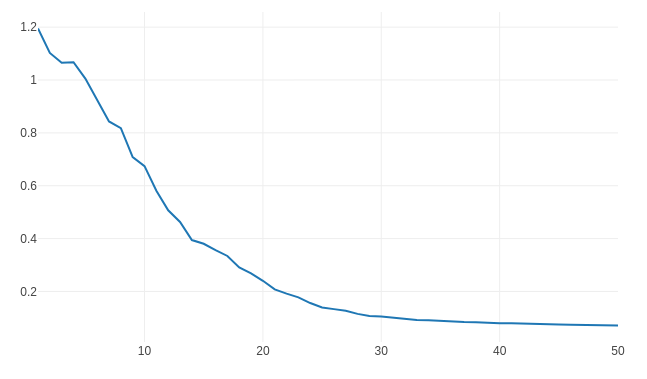The loss function decreases with the number of epochs
1. Let's see what the final accuracy of our model is:
`import numpy as npinputs = torch.autograd.Variable(torch.Tensor(test_input).float())targets = torch.autograd.Variable(torch.Tensor(test_target).long())optimizer.zero_grad()out = net(inputs)_, predicted = torch.max(out.data, 1)error_count = test_target.size - np.count_nonzero((targets == predicted).numpy())print('Errors: %d; Accuracy: %d%%' % (error_count, 100 * torch.sum(targets == predicted) / test_target.size))`

We do this by feeding the test set to the network and computing the error manually. The output is as follows:

`Errors: 0; Accuracy: 100%`

We were able to classify all 30 test samples correctly.

We must also keep in mind trying different hyperparameters of the network and see how the accuracy and loss functions work. You could try changing the number of units in the hidden layer, the number of epochs we train in the network, as well as the learning rate.

# Summary

In this chapter, we covered what machine learning is and why it's so important. We talked about the main classes of machine learning techniques and some of the most popular classic ML algorithms. We also introduced a particular type of machine learning algorithm, called neural networks, which is at the basis for deep learning. Then, we looked at a coding example where we used a popular machine learning library to solve a particular classification problem. In the next chapter, we'll cover neural networks in more detail and explore their theoretical justifications.

### About the Authors

• ##### Ivan Vasilev

Ivan Vasilev started working on the first open source Java deep learning library with GPU support in 2013. The library was acquired by a German company, where he continued to develop it. He has also worked as a machine learning engineer and researcher in the area of medical image classification and segmentation with deep neural networks. Since 2017, he has been focusing on financial machine learning. He is working on a Python-based platform that provides the infrastructure to rapidly experiment with different machine learning algorithms for algorithmic trading. Ivan holds an MSc degree in artificial intelligence from the University of Sofia, St. Kliment Ohridski.

Browse publications by this author
• ##### Daniel Slater

Daniel Slater started programming at age 11, developing mods for the id Software game Quake. His obsession led him to become a developer working in the gaming industry on the hit computer game series Championship Manager. He then moved into finance, working on risk- and high-performance messaging systems. He now is a staff engineer working on big data at Skimlinks to understand online user behavior. He spends his spare time training AI to beat computer games. He talks at tech conferences about deep learning and reinforcement learning; and the name of his blog is Daniel Slater's blog. His work in this field has been cited by Google.

Browse publications by this author
• ##### Gianmario Spacagna

Gianmario Spacagna is a senior data scientist at Pirelli, processing sensors and telemetry data for internet of things (IoT) and connected-vehicle applications. He works closely with tire mechanics, engineers, and business units to analyze and formulate hybrid, physics-driven, and data-driven automotive models. His main expertise is in building ML systems and end-to-end solutions for data products. He holds a master's degree in telematics from the Polytechnic of Turin, as well as one in software engineering of distributed systems from KTH, Stockholm. Prior to Pirelli, he worked in retail and business banking (Barclays), cyber security (Cisco), predictive marketing (AgilOne), and did some occasional freelancing.

Browse publications by this author
• ##### Peter Roelants

Peter Roelants holds a master's in computer science with a specialization in AI from KU Leuven. He works on applying deep learning to a variety of problems, such as spectral imaging, speech recognition, text understanding, and document information extraction. He currently works at Onfido as a team leader for the data extraction research team, focusing on data extraction from official documents.

Browse publications by this author
• ##### Valentino Zocca

Valentino Zocca has a PhD degree and graduated with a Laurea in mathematics from the University of Maryland, USA, and University of Rome, respectively, and spent a semester at the University of Warwick. He started working on high-tech projects of an advanced stereo 3D Earth visualization software with head tracking at Autometric, a company later bought by Boeing. There he developed many mathematical algorithms and predictive models, and using Hadoop he automated several satellite-imagery visualization programs. He has worked as an independent consultant at the U.S. Census Bureau, in the USA and in Italy. Currently, Valentino lives in New York and works as an independent consultant to a large financial company.

Browse publications by this author

### Latest Reviews

(3 reviews total)